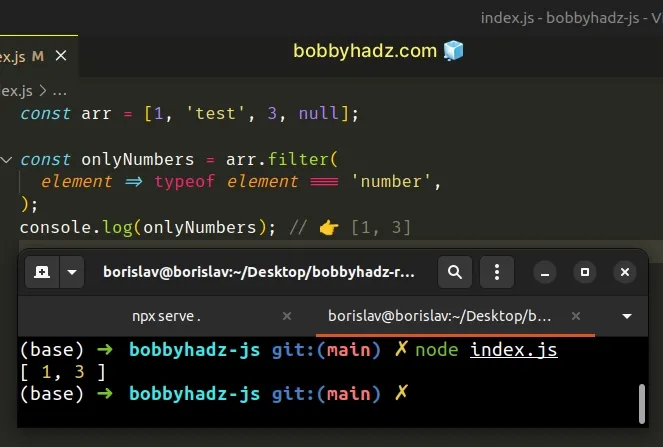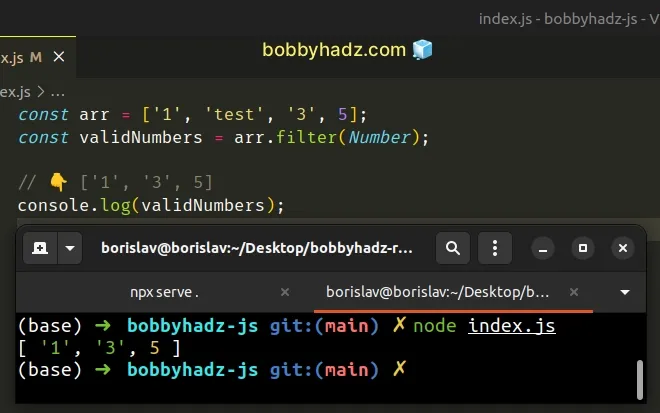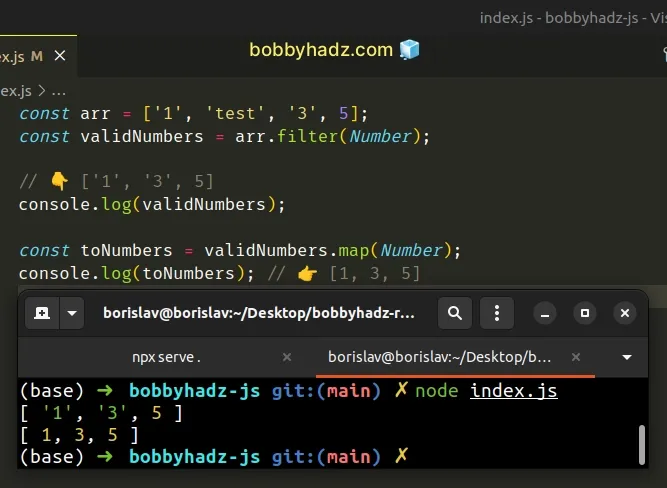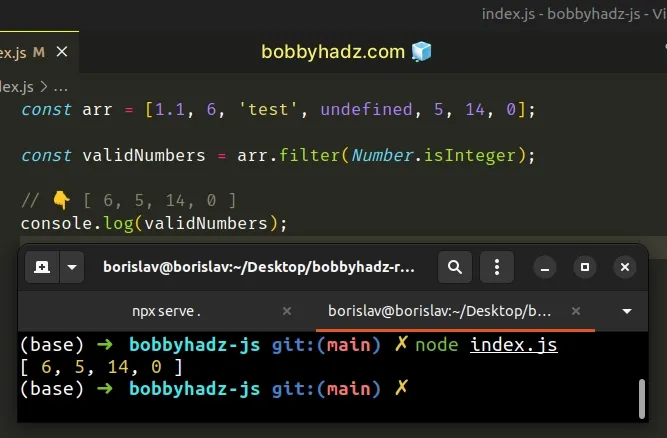# Filter an Array to only Numbers in JavaScriptLast updated: Jan 8, 2023
3 min## #Filter an Array to only Numbers in JavaScript

To filter an array to only numbers:

1. Use the `Array.filter()` method to iterate over the array.
2. Use the `typeof` operator to check if each element is a number.
3. The `filter` method will return a new array containing the numbers of the original array.
index.js
```Copied!```const arr = [1, 'test', 3, null];

const onlyNumbers = arr.filter(
element => typeof element === 'number'
);
console.log(onlyNumbers); // 👉️ [1, 3]
``````The function we passed to the Array.filter method gets called with each element in the array.

The `filter()` method returns a new array that only contains the elements that meet the condition.

On each iteration, we check if the element has a type of `number` using the typeof operator.

The `typeof` operator returns a string that indicates the type of a value.

All the elements that meet the condition (have a type of number) will get added to the new array.

## #Dealing with string elements that are valid numbers

If you have an array of strings that might be valid numbers, pass the `Number` object to the `filter()` method.

index.js
```Copied!```const arr = ['1', 'test', '3', 5];
const validNumbers = arr.filter(Number);

// 👇️ ['1', '3', 5]
console.log(validNumbers);
``````We used the `filter` method to iterate over the array. On each iteration, we convert the element to a number and return the result.

The `Number()` constructor takes the current element, converts it to a number and returns the result.

Note that if a value of `0` is passed to the number object, it wouldn't get included in the new array because `0` is a falsy value.

The falsy values in JavaScript are: `null`, `undefined`, `false`, `0`, `""` (empty string), `NaN` (not a number).

You can explicitly check for `0` to have zeros pass the test as well.

index.js
```Copied!```const arr = ['1', 'test', '3', 5, 0];

const validNumbers = arr.filter(element => {
return element === 0 || Number(element);
});

// 👇️ [ '1', '3', 5, 0 ]
console.log(validNumbers);
``````

We used the logical OR (||) operator, so for the element to be included in the new array, either condition has to be met.

## #Converting the results to numbers

The `filter` method doesn't convert the strings in the array to numbers, it simply returns the elements that meet the condition.

You can call the `map()` method on the filtered array to get an array of numbers.

index.js
```Copied!```const arr = ['1', 'test', '3', 5];
const validNumbers = arr.filter(Number);

// 👇️ ['1', '3', 5]
console.log(validNumbers);

const toNumbers = validNumbers.map(Number);
console.log(toNumbers); // 👉️ [1, 3, 5]
``````The function we passed to the Array.map() method gets called with each element in the array.

The `map()` method returns a new array containing the values returned from the callback function.

## #Filter an Array to only Integers in JavaScript

To filter an array to only integers:

1. Use the `Array.filter()` method to iterate over the array.
2. Use the `Number.isInteger()` method to check if each element is an integer.
3. The `filter()` method will return a new array containing only the integers.
index.js
```Copied!```const arr = [1.1, 6, 'test', undefined, 5, 14, 0];

const validNumbers = arr.filter(Number.isInteger);

// 👇️ [ 6, 5, 14, 0 ]
console.log(validNumbers);
``````The Number.isInteger() method returns `true` if the provided value is an integer and `false` otherwise.

Here are some examples of using the `Number.isInteger()` method directly.

index.js
```Copied!```console.log(Number.isInteger(1)); // 👉️ true
console.log(Number.isInteger(1.5)); // 👉️ false
console.log(Number.isInteger('1')); // 👉️ false
console.log(Number.isInteger(-1)); // 👉️ true
``````

On each iteration, we check if the current element is an integer and return the result.

The new array contains only the integer values of the original array.